Submit a blog to KDnuggets -- Top Blogs Win A Reward

Topics: AI | Data Science | Data Visualization | Deep Learning | Machine Learning | NLP | Python | R | Statistics

KDnuggets Home » News » 2019 » Sep » Tutorials, Overviews » Ensemble Methods for Machine Learning: AdaBoost ( 19:n35 )

# Ensemble Methods for Machine Learning: AdaBoost

It turned out that, if we ask the weak algorithm to create a whole bunch of classifiers (all weak for definition), and then combine them all, what may figure out is a stronger classifier.

By Valentina Alto, MSc in Data Science and Business AnalyticsIn statistics and machine learning, ensemble methods use multiple learning algorithms to obtain better predictive performance than could not be obtained from any of the constituent learning algorithms alone.

The idea of combining multiple algorithms was first developed by computer scientist and Professor Michael Kerns, who was wondering whether “weakly learnability is equivalent to strong learnability “. The goal was turning a weak algorithm, barely better than random guessing, into a strong learning algorithm. It turned out that, if we ask the weak algorithm to create a whole bunch of classifiers (all weak for definition), and then combine them all, what may figure out is a stronger classifier.

AdaBoost, which stays for ‘Adaptive Boosting’, is a machine learning meta-algorithm which can be used in conjunction with many other types of learning algorithms to improve performance.

In this article, I’m going to provide an idea of the maths behind Adaboost, plus I’ll provide an implementation in Python.

### Intuition and Maths behind AdaBoost

Imagine we have our sample, divided into a training set and test set, and a bunch of classifiers. Each of them is trained on a random subset of the training set (note that those subsets can actually overlap-it is not the same as, for example, cross-validation). Then, for each observation in each subset, AdaBoost assigns a weight, which determines the probability that this observation will appear in the training set. Observations with higher weights are more likely to be included in the training set. Hence, AdaBoost tends to assign higher weights to those observations which have been misclassified, so that they will represent a larger part of the next classifiers training set, with the aim that, this time, the next classifier trained will perform better on them.

Considering a simple binary classification problem, whose targets are represented by the signs ‘positive’ or ‘negative’ (expressed as 1 and -1), the equation of the final classifier looks like that: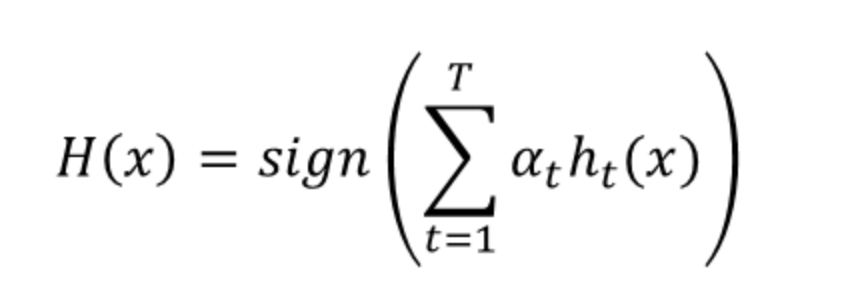Basically, the output of the final classifier for observation x is equal to sign of the weighted sum of the outputs h_t(x) of T weak classifiers, with weights equal to α_t.

More specifically, α_t is the weight assigned to the output of classifier t (note that this weight is different from that which will be assigned to observations, which will be discussed later on). It is computed as follows: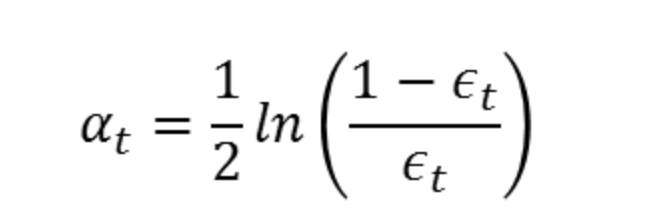Where ε_t is the error term of classifier t (misclassified observations/total observations). Of course, classifiers with low error will be prioritized in the sum, hence their weight will be higher. Indeed, if we look at the alpha corresponding to different errors:

```import numpy as np
import matplotlib.pyplot as plt

epsilon=np.arange(0,1,0.01)
alpha=1/2*np.log((1-epsilon)/epsilon)

plt.plot(epsilon, alpha)
plt.xlabel('error')
plt.ylabel('alpha')```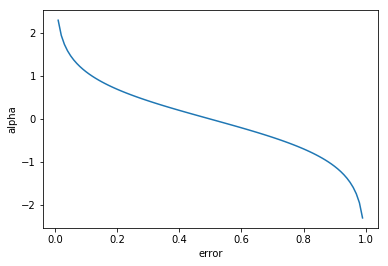As you can see, the classifier’s weight grows exponentially as the error approaches 0, while it grows exponentially negative as the error approaches 1.

The value of alpha is then used to compute the other type of weights, those that will be attributed to the observations of the subset. For the first classifier, the weights are equally initialized, so that each observation has a weight=1/n (where n=size of the subset). From the second classifier, each weight is recursively computed as: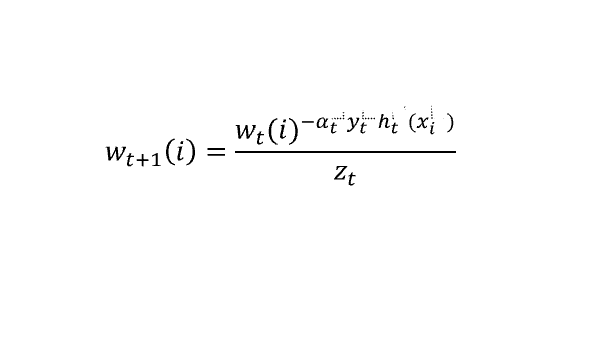Where y_t is the target value (either 1 or -1) and the variable w_t is a vector of weights, with one weight for each training example in the training set. ‘i’ is the training example number. This equation shows you how to update the weight for the ith training example.

We can look at w_t as a distribution: it is consistent with what we said at the beginning, that is, weights represent the probability that training example will be selected as part of the training set.

To make it a distribution, all of these probabilities should add up to 1. To ensure this, we normalize the weights by dividing each of them by the sum of all the weights, Z_t.

Let’s interpret this formula. Since we are dealing with binary classification (-1 vs 1), the product y_t*h_t(x_i) will be positive if both the actual and fitted values have the same sign (well-classified), negative if they have different signs (misclassified). Hence:

• if the product is positive and alpha is greater than zero (strong classifier), the weight attributed to the ith observation will be small
• if the product is positive and alpha is less than zero (weak classifier), the weight attributed to the ith observation will be high
• if the product is negative and alpha is greater than zero (strong classifier), the weight attributed to the ith observation will be high
• if the product is negative and alpha is less than zero (weak classifier), the weight attributed to the ith observation will be small

Note: when I say ‘small’ and ‘high’ I’m mean, if we consider the exponential before normalization, respectively less than 1 and greater than 1. Indeed: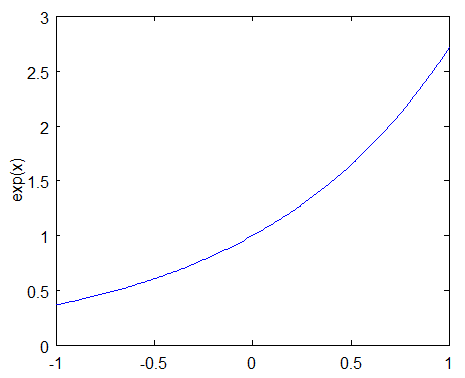Now that we have an idea of how AdaBoost works, let’s see its implementation in Python using the well-known Iris Dataset.

### Implementation with Python

Let’s first import our data:

```from sklearn.ensemble import AdaBoostClassifier
from sklearn import datasets
from sklearn.model_selection import train_test_split
from sklearn import metrics

X = iris.data
y = iris.target

X_train, X_test, y_train, y_test = train_test_split(X, y, test_size=0.3)```

The default algorithm in AdaBoost is decision tree, but you can decide to manually set a different classifier. Here, I’m going to use the Support Vector Machine classifier (you can read more about SVM here).

```from sklearn.svm import SVC
from sklearn import metrics
svc=SVC(probability=True, kernel='linear')

model = abc.fit(X_train, y_train)

y_pred = model.predict(X_test)
print("Accuracy:",metrics.accuracy_score(y_test, y_pred))

Output:
Accuracy: 0.9777777777777777```

As you can see, almost 98% of our observations have been correctly classified.

### Conclusions

AdaBoost is a technique easy to implement. It iteratively corrects the mistakes of the weak classifier improving accuracy. However, it is not free from caveats. Indeed, since it looks for a reduction in the training error, it is particularly sensitive to outliers, with the risk of producing an overfitted model which won’t fit new, unlabeled data well, since it lacks of generalization (you can read more about overfitting here).

Originally published at http://datasciencechalktalk.com on September 7, 2019.

Bio: Valentina Alto is a Machine Learning and Statistics enthusiast, currently pursuing a MSc in Data Science at Bocconi University.

Original. Reposted with permission.

Related:

## Top Stories Past 30 Days

Most Popular
Most SharedGet KDnuggets, a leading newsletter on AI, Data Science, and Machine Learning